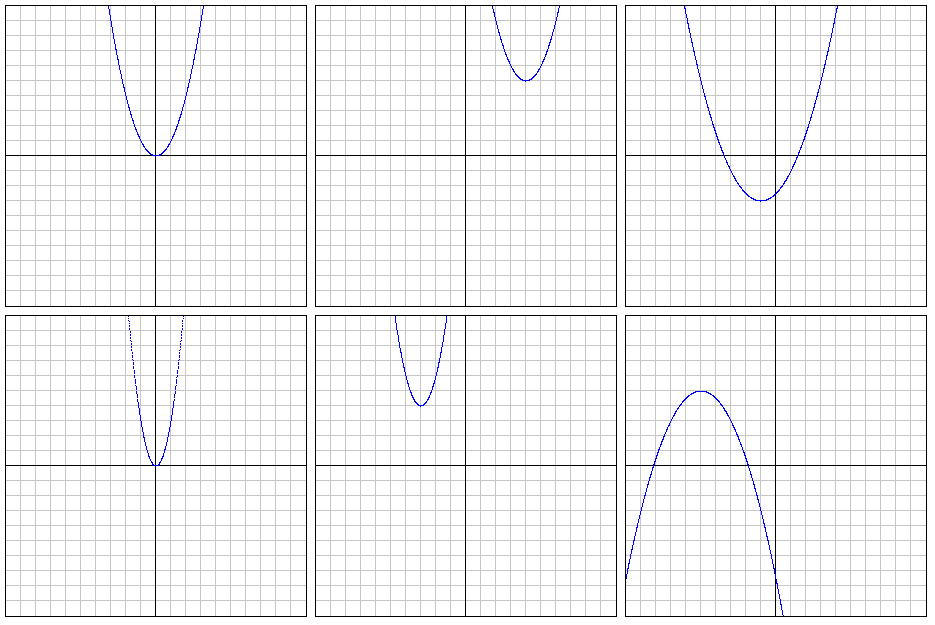• Author: Graham Mitchell
• Filename: GraphingParabolas.java

### Graphing Parabolas

These exercises allow you to practice graphing using for loops.

#### Files needed:

Write code to reproduce, as accurately as possible, the following six parabolas.

Remember that the standard form of a parabola is `y = a(x-h)² + k`
where the vertex is at the point `(h,k)`, and the value of `a` influences the direction and width.```
```